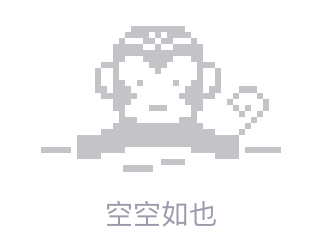# 当某些特定值与给定数组匹配时，从多维数组中删除键

I'm sure I'm only facing a logical problem here.

I have an array like this :

``````var_export(\$myarray);

array (
0 =>
array (
'inf:name' => 'aaa',
'inf:value' => '111',
'inf:children' => NULL,
),
1 =>
array (
'inf:name' => 'bbb',
'inf:value' => '222',
'inf:children' => NULL,
),
2 =>
array (
'inf:name' => 'ccc',
'inf:value' => '333',
'inf:children' => NULL,
),
3 =>
array (
'inf:name' => 'bob',
'inf:children' =>
array (
0 =>
array (
'inf:name' => 'ddd',
'inf:value' => '444',
'inf:children' => NULL,
),
),
),
4 =>
array (
'inf:name' => 'data',
'inf:children' =>
array (
0 =>
array (
'inf:name' => 'eee',
'inf:value' => '555',
'inf:children' => NULL,
),
1 =>
array (
'inf:name' => 'steve',
'inf:value' => 'bar1',
'inf:children' => NULL,
),
2 =>
array (
'inf:name' => 'john',
'inf:value' => 'bar2',
'inf:children' => NULL,
),
3 =>
array (
'inf:name' => 'peter',
'inf:value' => 'bar3',
'inf:children' =>
array (
0 =>
array (
'inf:name' => 'fff',
'inf:value' => '666',
'inf:children' => NULL,
),
),
),
),
),
)

//Or using print_r()
print_r(\$myarray);

Array
(
 => Array
(
[inf:name] => aaa
[inf:value] => 111
[inf:children] =>
)

 => Array
(
[inf:name] => bbb
[inf:value] => 222
[inf:children] =>
)

 => Array
(
[inf:name] => ccc
[inf:value] => 333
[inf:children] =>
)

 => Array
(
[inf:name] => bob
[inf:children] => Array
(
 => Array
(
[inf:name] => ddd
[inf:value] => 444
[inf:children] =>
)

)

)

 => Array
(
[inf:name] => data
[inf:children] => Array
(
 => Array
(
[inf:name] => eee
[inf:value] => 555
[inf:children] =>
)

 => Array
(
[inf:name] => steve
[inf:value] => bar1
[inf:children] =>
)

 => Array
(
[inf:name] => john
[inf:value] => bar2
[inf:children] =>
)

 => Array
(
[inf:name] => peter
[inf:value] => bar3
[inf:children] => Array
(
 => Array
(
[inf:name] => fff
[inf:value] => 666
[inf:children] =>
)

)

)

)

)
)
``````

I would like to remove some keys when `inf:name` matches the given names.

Here is my test function :

``````function array_cleanup( \$array, \$todelete )
{
foreach( \$array as \$key => \$value )
{
if( is_array( \$value ) )
{
\$array[\$key] = array_cleanup( \$array[ \$key ], \$todelete );
} else {
if( sizeOf( \$todelete ) > 0 )
{
if ( in_array( \$value[ 'inf:name' ], \$todelete ) )
unset( \$array[ \$key ] );
}
}
}
return \$array;
}

\$newarray = array_cleanup( \$myarray, array("aaa", "peter", "ccc") );
``````

The desired output must be :

``````var_dump(\$newarray);

array (
0 =>
array (
'inf:name' => 'bbb',
'inf:value' => '222',
'inf:children' => NULL,
),
2 =>
array (
'inf:name' => 'bob',
'inf:children' =>
array (
0 =>
array (
'inf:name' => 'ddd',
'inf:value' => '444',
'inf:children' => NULL,
),
),
),
4 =>
array (
'inf:name' => 'data',
'inf:children' =>
array (
0 =>
array (
'inf:name' => 'eee',
'inf:value' => '555',
'inf:children' => NULL,
),
1 =>
array (
'inf:name' => 'steve',
'inf:value' => 'bar1',
'inf:children' => NULL,
),
2 =>
array (
'inf:name' => 'john',
'inf:value' => 'bar2',
'inf:children' => NULL,
),
),
),
)

//or using print_r();
print_r(\$newarray);
Array
(
 => Array
(
[inf:name] => bbb
[inf:value] => 222
[inf:children] =>
)

 => Array
(
[inf:name] => bob
[inf:children] => Array
(
 => Array
(
[inf:name] => ddd
[inf:value] => 444
[inf:children] =>
)

)

)

 => Array
(
[inf:name] => data
[inf:children] => Array
(
 => Array
(
[inf:name] => eee
[inf:value] => 555
[inf:children] =>
)

 => Array
(
[inf:name] => steve
[inf:value] => bar1
[inf:children] =>
)

 => Array
(
[inf:name] => john
[inf:value] => bar2
[inf:children] =>
)

)

)
)
``````

The `\$newarray` keys must be reset. Because I might face other problems later if they aren't reset.

Thank you guys.2010/12/15 15:52
• php
• 点赞
• 收藏
• 回答
私信

#### 2个回复